Latest Banking jobs   »   IBPS quant

# Quantitative Aptitude Quiz For IBPS RRB Clerk Mains 2023-15th-September

Directions (1-5): The following questions are accompanied by two statements (I) and (II). You have to determine which statements(s) is/are sufficient/necessary to answer the questions.
(a) Statement (I) alone is sufficient to answer the question but statement (II) alone is not sufficient to answer the question.
(b) Statement (II) alone is sufficient to answer the question but statement (I) alone is not sufficient to answer the question.
(c) Both the statements taken together are necessary to answer the question, but neither of the statements alone is sufficient to answer the question.
(d) Either statement (I) or statement (II) by itself is sufficient to answer the question.
(e) Statements (I) and (II) taken together are not sufficient to answer the question.

IBPS RRB Clerk Mains Exam Analysis 2023

Q1. An article is marked 35% above its cost price, find the cost price of the article.
Statement I: Discount allowed on article is Rs.190 more than profit earned on the article.
Statement II: Ratio of selling price of the article to marked price of the article is 4 : 5.

Q2. Deepak is 20% less efficient than Dharam. Find efficiency of Shivam.
Statement I: Shivam and Dharam working together can complete a piece of work in 26 2/3 days and Deepak and Shivam working together an complete same piece of work in 28 4/7 days.
Statement II: Deepak is 60% less efficient than Shivam and Dharam working alone can complete a piece of work in 80 days.

Q3. Asif invested Rs.80000 across two schemes – A & B offering SI in the ratio 5 : 3 respectively. Find total interest earned by him.
Statement I: Rate of interest offered by scheme – B is twice of that of offered by scheme – A and period of investment in both schemes is 4 years.
Statement II: Interest received from scheme – B is Rs.6000 more than interest received from scheme – A.

Q4. Average of present age of Aman, Bhanu and Chaman is 34 years. Find present age of Chaman.
Statement I: Aman’s present age is 100% more than Chaman’s present age and ratio of Bhanu’s age 6 years hence to Chaman’s present age is 2 : 1.
Statement II: Sum of Aman’s and Bhanu’s present age is 78 years.

Q5. Ratio of length of train – A and train – B is 4 : 5. Find speed of train – A.
Statement I: Train – A can cross a 500m long platform in 28 seconds and train – A crosses train – B while running in same direction in 54 seconds.
Statement II: Train – B can cross a pole in 15 seconds.

Directions (6-10): The following questions are accompanied by two statements A and B. You have to determine which statements(s) is/are sufficient/necessary to answer the questions.
(a) Statement A alone is sufficient to answer the question but statement B alone is not sufficient to answer the questions.
(b) Statement B alone is sufficient to answer the question but statement A alone is not sufficient to answer the question.
(c) Both the statements taken together are necessary to answer the questions, but neither of the statements alone is sufficient to answer the question.
(d) Either statement A or statement B by itself is sufficient to answer the question.
(e) Statements A and B taken together are not sufficient to answer the question.

Q6. Find the overall profit or loss percentage of retailer if he sells two bats and four balls.
(A) Cost price of 3 bats is equal to selling price of 4 balls while profit percentage on selling one bat is 50%.
(B) On selling two bats retailer earn 50% profit while on selling four balls retailers’ loss 40% of his money.

Q7. A box contains 4 green ball, ‘x’ blue balls, 5 violet balls and ‘y’ yellow balls. Find the value of ‘x + y’.
(A) Probability of choosing two green ball is 1/20.
(B) Probability of choosing one blue ball or one violet ball is ½.

Q8. If w > 1 and n ≠ 0,1 and ‘w’ and ‘n’ both are integers. Is ‘n’ a positive integer?
(A) w^2n>w^n
(B) n^2w > n^w

Q9. Find the number of persons in a group if average weight of the group is 16?
(A) If one person left the group having weight 16 then average weight of the group is same as before.
(B) If two persons join the group having weight 16 and 28, then the average weight of group increases by 2.

Q10. In how many days ‘A’, ‘B’ and ‘C’ together can complete the work?
(A) A is 50% more efficient then B who is 100% more efficient than C while A and B together can complete the work in 12 days.
(B) ‘A’ and ‘B’, ‘B’ and ‘C’ and ‘C’ and ‘A’ together can complete the work in 12, 20 and 15 days respectively.

Solutions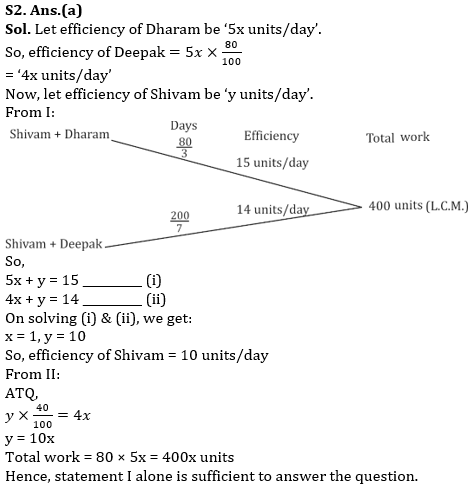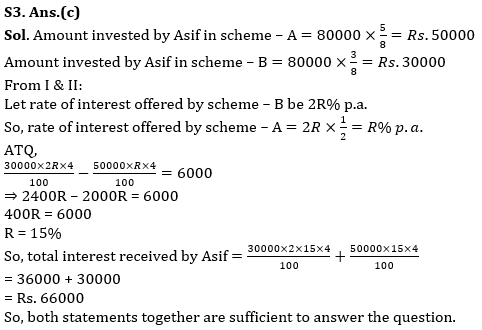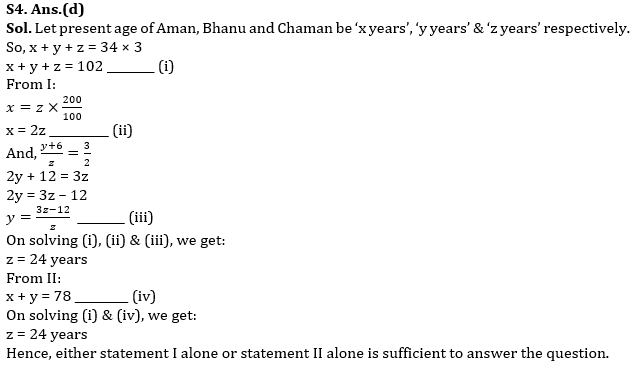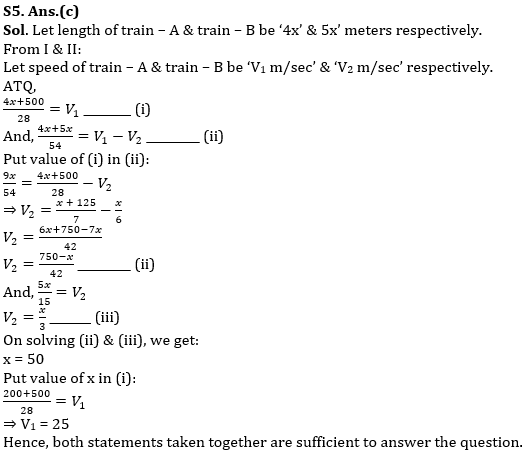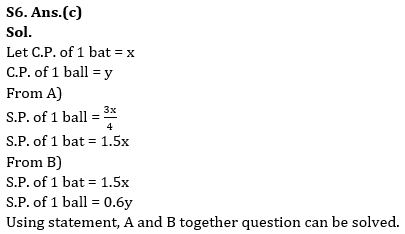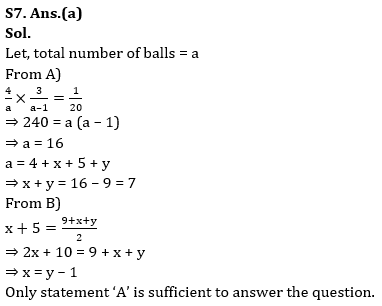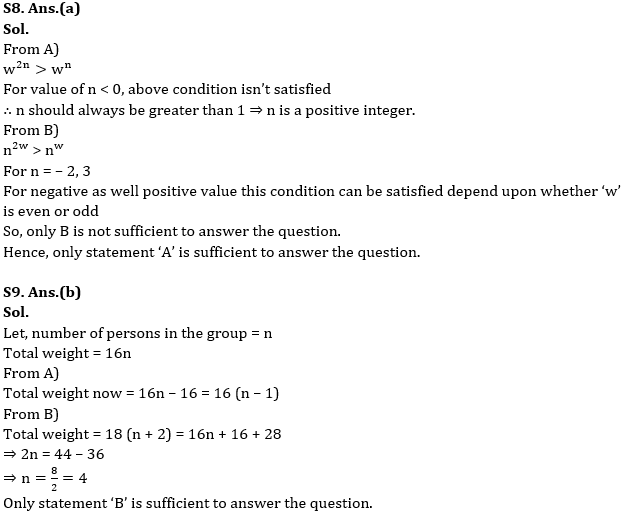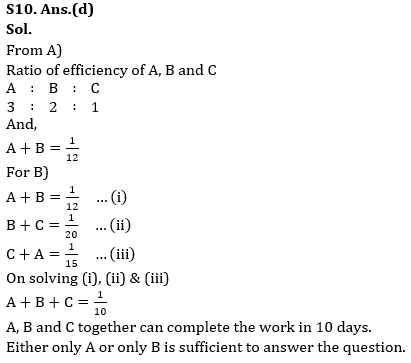## FAQs

### When will the IBPS RRB Clerk Mains Exam be conducted?

IBPS RRB Clerk Mains Exam will be conducted on 16 September 2023.

#### Congratulations!Union Budget 2023-24: Free PDF